Function Repository Resource:

# PolynomialDegree

Compute the degree of a polynomial in any number of variables

Contributed by: Dennis M Schneider
 ResourceFunction["PolynomialDegree"][poly,vars] returns the degree of a polynomial in vars.

## Details and Options

The second argument vars can be a list of any number of variables.

## Examples

### Basic Examples (4)

Compute the degree of a basic polynomial expression:

 In:=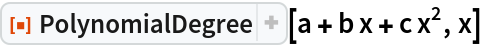Out=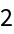Compute the degree of a polynomial in two variables:

 In:=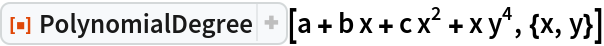Out=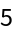The following expression is constant with respect to the variables z, w, u:

 In:=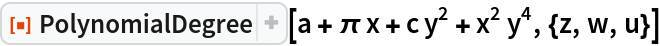Out=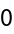The following expression is not a polynomial in x and y:

 In:=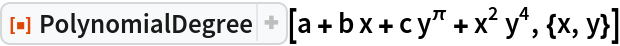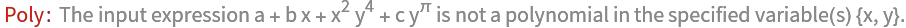### Properties and Relations (1)

A constant polynomial has degree 0 but the zero polynomial (even though it is a constant polynomial) does not have a degree (though some authors assign it to have degree -1 or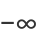):

 In:=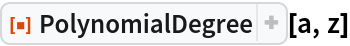Out=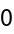In:=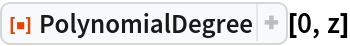Out=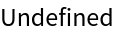## Publisher

Dennis M Schneider

## Version History

• 1.0.0 – 30 July 2019